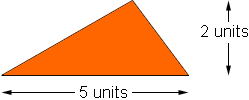How do you find the area of a equalateral and other triangles? Phill Student middle or secondary class thank you Hi Phill, The most common expression used to find the area of a triangle is  1/2 x base x height. Thus, for example, the triangle below has a base of 5 units and a height of 2 units so its area is  1/2 x 5 x 2 = 5 square units.A second expression that is often useful is Herron's Formula. You can find the formula and an example in the answer to a previous question. Penny Go to Math Central To return to the previous page use your browser's back button.